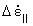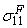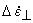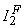U.S. Department of Transportation
1200 New Jersey Avenue, SE
Washington, DC 20590
202-366-4000

Federal Highway Administration Research and Technology
Coordinating, Developing, and Delivering Highway Transportation InnovationsThis report is an archived publication and may contain dated technical, contact, and link information
 Federal Highway Administration > Publications > Research Publications > 04097 > Measured Variability Of Southern Yellow Pine - Manual for LS-DYNA Wood Material Model 143
Publication Number: FHWA-HRT-04-097
Date: August 2007

# Measured Variability Of Southern Yellow Pine - Manual for LS-DYNA Wood Material Model 143

PDF files can be viewed with the Acrobat® Reader®

### 1.6 HARDENING

Wood exhibits prepeak nonlinearity in compression parallel and perpendicular to the grain. Perpendicular hardening was previously demonstrated in figure 3(b) for clear pine. A choice was made between two possible approaches for modeling prepeak nonlinearity. The first approach was to model bilinear hardening behavior by specifying initial and hardening moduli. This approach simulates a sudden change in modulus. However, real data, such as that previously shown in figure 3(b), exhibits a gradual change in modulus. The second approach was to model a translating yield surface that simulates a gradual change in modulus. This is the chosen approach.

#### 1.6.1 Model Overview

Our approach is to define initial yield surfaces that harden (translate) until they coincide with the ultimate yield surfaces, as demonstrated in figure 13 for the parallel modes. Separate formulations are modeled for the parallel and perpendicular modes. The initial location of the yield surface determines the onset of plasticity. The rate of translation determines the extent of nonlinearity.

Figure 13.

Prepeak nonlinearity is modeled in compression with translating yield surfaces that allow user to specify the hardening response.

For each mode (parallel and perpendicular), two hardening parameters must be defined. The first parameter, N, determines the onset of nonlinearity of the load-deflection or stress-strain curves. For example, consider the case where the user wants prepeak nonlinearity to initiate at 60 percent of the peak strength. The user inputs = 0.4 so that 1 – N = 0.6. The second parameter, c, determines the rate of hardening(i.e.,how rapidly or gradually the load-deflection or stress-strain curves harden). If the user wants rapid hardening, then a large value of c is input (e.g., c = 1000). If the user wants gradual hardening, then a small value of c is input (e.g., c = 10). The value of c needed for any particular fit depends on the properties of the material being modeled. It is selected by running simulations (single-element simulations are fastest) with various values of c and comparing those simulations with data. Hardening model equations are given in section 1.6.3.

In addition to modeling prepeak nonlinearity as shown in figure 13, a separate formulation models postpeak hardening, as shown in figure 14. Instead of coinciding with the ultimate yield surface, the initial yield surface passes through the ultimate yield surface. The larger the value of the input parameter Ghard,the more pronounced the postpeak hardening. A zero value for Ghard will produce perfectly plastic behavior. The default value is zero. Currently, Ghard controls both the parallel and perpendicular behaviors simultaneously.

Figure 14.

Postpeak hardening is modeled in compression with positive values of the parameter Ghard.

#### 1.6.2 Default Hardening Parameters

Default hardening parameters for clear wood pine and fir are given in table 7. These hardening parameters were set by the contractor by correlating LS-DYNA simulations with compression tests conducted on 15- by 15- by 305-centimeter (cm) timbers.(2) Values of N|| = 0.5 and N^ = 0.4 were fit to the timber compression data for parallel and perpendicular behavior, respectively. These values for N|| and N^ are independent of grade, temperature, and moisture content. Values for c|| and c^ depend on the wood grade, but are independent of temperature and moisture content. Good fits to the data were obtained with the following formulas:

Qc = compression strength-reduction factor for graded wood discussed in section 1.12.

Table 7. Default hardening parameters for clear wood southern yellow pine and Douglas fir.
Wood Parallel Perpendicular
N|| c|| N^ c^
Southern Yellow Pine
0.5
400
0.4
100
Douglas Fir
0.5
400
0.4
100

#### 1.6.3 Hardening Model Theory

Parallel Modes

The state variable that defines the translation of the yield surface is known as the backstress and is denoted by aij. Prepeak nonlinearity is modeled in compression, but not shear, so the only backstress required for the parallel modes is a11. The value of the backstress is a11 = 0 upon initial yield and a11 = –N||Xc at ultimate yield (in uniaxial compression). The maximum backstress occurs at ultimate yield and is equal to the total translation of the yield surface in stress space.

The hardening rule defines the growth of the backstress. Hardening rules are typically based on stress or plastic strain. Hardening is based on stress. This is accomplished by defining the incremental backstress:

where:
c||  = user input parameter that determines rate of translation
G||  = function that properly limits the increments
s11a11  = reduced stress that determines direction of translation (longitudinal)= effective strain-rate increment parallel to the grain
Dt  = time step

These terms are internally calculated by the material model and LS-DYNA, and are included to keep the hardening response independent of time step, time-step scale factor, and strain increment. The effective strain rate is a scalar value that is equal to:

The function G|| restricts the motion of the yield surface so that it cannot translate outside the ultimate surface.(20) The functional form of G|| is determined from the functional form of the yield surface and the longitudinal stress definition. A brief derivation is given in appendix E. Thus, it is defined as:

The value of the limiting function is G||= 1 at initial yield (because a11 = 0) and G||= 0 at ultimate yield (because a11 = N||). Thus, G|| limits the growth of the backstress as the ultimate surface is approached. If postpeak hardening is active, then the minimum value is maintained at G||= Ghard rather than G||= 0. The ultimate yield surface is defined from equation 13 as:

For the case of uniaxial compressive stress (no shear), the ultimate yield surface reduces to= Xc.

Perpendicular Modes

Prepeak nonlinearity is modeled in compression, but not shear, so the backstress components required are a22 and a33. The value of the backstress sum is a22 + a33 = 0 upon initial yield and a22 + a33 = –N^Yc at ultimate yield (biaxial compression without shear). The backstress increments are defined as follows:

where:
c^  =  user input parameter that determines rate of translation=  effective strain-rate increment perpendicular to the grain:

The functional form of G^ is determined from the functional form of the yield surface in equation 14 as:

The value of G^ ranges from 1 at initial yield to 0 at ultimate yield. If postpeak hardening is active, then the minimum value is G^= Ghard rather than G^= 0. The ultimate yield surface is defined from equation 14 as:

Consider the case of biaxial compressive stress (I3 = 0). Initially, a22 + a33 = 0, so G^ = 1. Ultimately, a22 + a33 = –N^Yc and= s22 + s33 = Yc, so G^ = 0.

#### 1.6.4 Implementation Aspects

The plasticity model discussed in the section 1.5 is modified to account for compressive hardening. Modifications are made to the failure surface definitions and the stress updates.

Failure Surface Definitions With Hardening

For the parallel modes, initial yielding occurs when f|| > 0, with:

For the perpendicular modes, initial yielding occurs when f^ > 0, with:

No modifications are necessary for the tensile modes.